##### SAT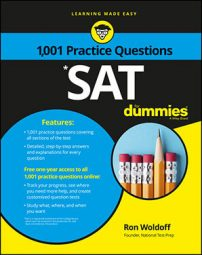If you encounter a question on the SAT Math exam that gives you the equation of a circle, you'll probably need to convert that equation to the standard circle equation.

The following practice questions give you the equation of a circle and ask you to find its radius and center.

## Practice questions

Questions 1 and 2 are based on the following information.

The equation of a circle in the xy-plane is shown here:

x2 + y2 + 6x – 4y = –9

1. What is the radius of the circle? A. 1 B. 2 C. 3 D. 4
2. What are the (x, y) coordinates of the center? A. (–3, 2) B. (–2, 3) C. (3, –2) D. (2, –3)

1. The correct answer is Choice (B). First convert the equation to the standard circle equation: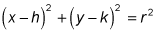where r is the radius of the circle. From the original equation, start by moving the x's and y's together: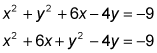The x2 + 6x tells you that (x + 3)2 is part of the equation. FOIL this out to x2 + 6x + 9. However, the x2 + 6x is by itself on the left, so add 9 to both sides of the equation: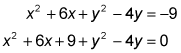Also, y2 – 4y tells you that (y – 2)2 is part of the equation, which FOILs out to y2 – 4y + 4. However, the y2 – 4y is by itself on the left, so add 4 to both sides, like this: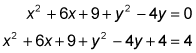To convert the circle to its standard form, factor x2 + 6x + 9 into (x + 3)2 and y2 – 4y + 4 into (y – 2)2, like this: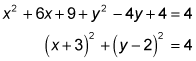Now the circle is in its familiar form, and r2 = 4, so r = 2.
2. The correct answer is Choice (A). First convert the equation to the standard circle equation:where h is the x-coordinate and k is the y-coordinate of the center of the circle. From the original equation, start by moving the x's and y's together: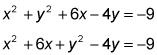The x2 + 6x tells you that (x + 3)2 is part of the equation. FOIL this out to x2 + 6x + 9. However, the x2 + 6x is by itself on the left, so add 9 to both sides of the equation:Also, y2 – 4y tells you that (y – 2)2 is part of the equation, which FOILs out to y2 – 4y + 4. However, the y2 – 4y is by itself on the left, so add 4 to both sides, like this:To convert the circle to its standard form, factor x2 + 6x + 9 into (x + 3)2 and y2 – 4y + 4 into (y – 2)2, like this:Now the circle is in its familiar form, where h = –3 and k = 2.